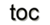Next: 8.3 Diffusion Simulation Up: 8. Generic Application Design Previous: 8.1 Visual Programming

# 8.2 Wave Equation

An example using the discrete concepts of electromagnetics in conjunction with the comprehensive operations of the GSSEis provided. It translates the continuous formulation of Maxwell's equations given in Section 1.2 with internally oriented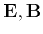and externally orientedfields, including their linking relations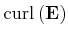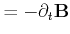(8.1)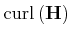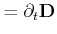(8.2)

into a discrete setting, by applying the discrete concepts of chains and cochains (Section 1.4 and Section 1.7). Figure 8.4 presents the discrete form of Faraday's and Amperé's law.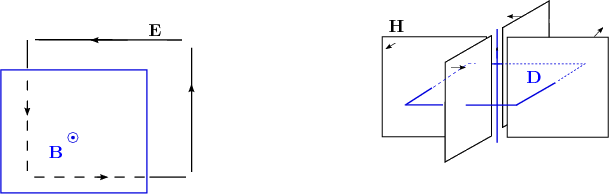Using a projection of the averaged field components onto 2-cells, local representatives of the global quantities are obtained. See Section 2.4.3 for more details. The following equation expresses this fact: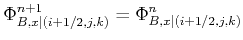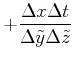(8.3)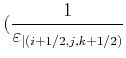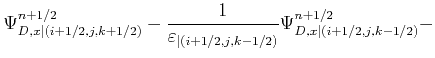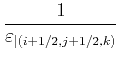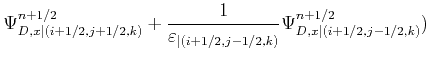By utilizing the quantity accessor mechanisms and the traversal operations of the GSSE, the equation can be rewritten as a discrete formulation, where the the electric field quantity is located on edges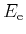and the magnetic field quantity is located on facets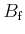. The permittivity, permeability, and spatial resolution are omitted to emphasize the topological traversal capabilities: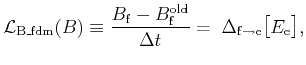(8.4)

where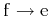denotes the traversal of all edges incident to a face. The topological traversal mechanism is presented in the following code snippet, where the constitutive laws are used to interpolate the corresponding quantities.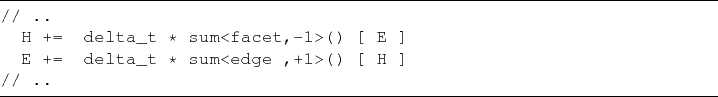The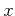-component and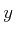-component of the final vector field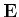is depicted in Figures 8.5-8.6 for a three-dimensional calculation. The x-y plane with a spatial dimension of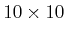units at the bottom uses a simple harmonically oscillating quantity and is also used as a Dirichlet contact. Neumann boundary conditions are applied to the remaining boundary planes.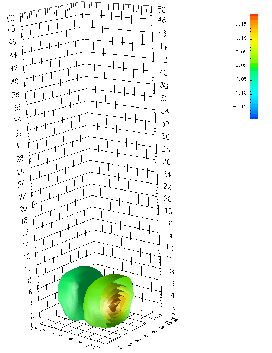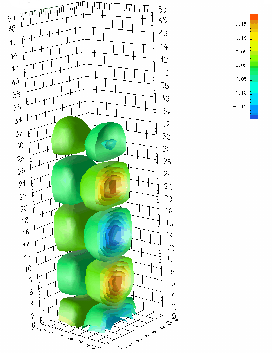Figure 8.5: Illustration of the-component ofwith a harmonic oscillating source in the x-y plane at two different time steps.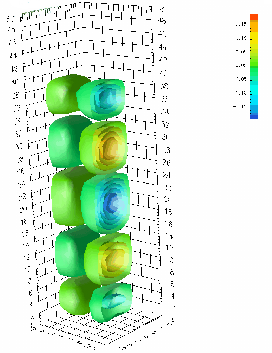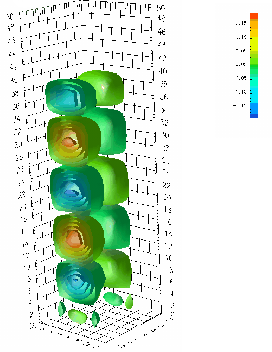Figure 8.6: Wave equation with a harmonic oscillating source in the x-y plane where the source is switched off. The-component ofis depicted on the right side.Next: 8.3 Diffusion Simulation Up: 8. Generic Application Design Previous: 8.1 Visual Programming

R. Heinzl: Concepts for Scientific Computing